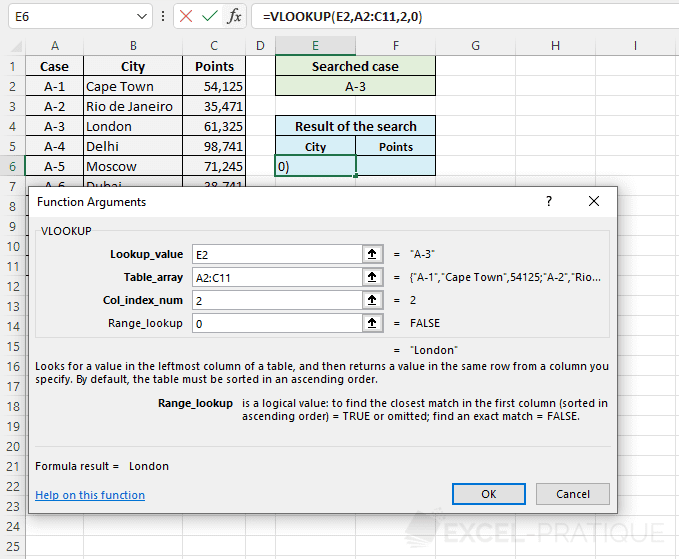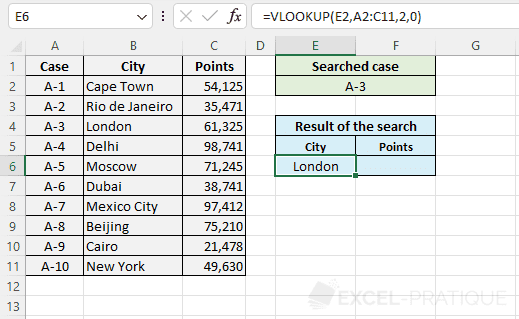# Excel function VLOOKUP

The VLOOKUP function searches for a value in the first column of a table and then returns the contents of the cell located on the same row as the lookup value.

Let's try an example:

We want to return a candidate's first name based on his number.

In this case, we are looking for candidate 8's first name.

• In the "Lookup_value" field, we enter G2, which contains the number 8.
• In the "Table_array" field, we define a data range that contains the table.
• In the "Col_index_num" field, we should enter the number of the column that contains the data to display (first names, in this case). The column number refers to the number of the column within the specified array.
• "Range_lookup" is optional, and allows you to either search for the exact value of the "Lookup_value" (by entering: FALSE), or to find the closest value to the "Lookup_value" (by entering: TRUE or leaving the field empty).And the result is the first name of candidate 8.

Now, in order to search for another name, all we have to do is edit the contents of cell G2.